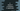# C program to check if a character is uppercase without using a library function## C program to check if a character is uppercase or not without using a library function:

In this post, we will learn how to check if a character is uppercase or not without using a library function. This program will take one character as input from the user, store it in a char variable and print one message.

I will show you two different ways to do that with examples.

### By comparing it with A and Z:

We can compare two characters by using >= or <= operators. So, if we check if the character is greater than or equal to A and less than or equal to Z, then it is an Uppercase character. Else it is not.

The below program does that:

``````#include <stdio.h>

int main() {
int i;
char givenValues = {'a','E','i','O','U', '3'};

for(i = 0; i< 6; i++){
if(givenValues[i] >= 'A' && givenValues[i] <= 'Z'){
printf("%c is an uppercase letter\n", givenValues[i]);
}else if(givenValues[i] >= 'a' && givenValues[i] <= 'z'){
printf("%c is a lowercase letter\n", givenValues[i]);
}else{
printf("%c is a not an alphabet letter\n", givenValues[i]);
}
}
return 0;
}``````

Here,

• givenValues is an array of characters. This array holds six different characters.
• We are using one for loop and iterating through the characters of givenValues array one by one.
• The if block checks if the current character is an uppercase character or not by comparing it with A and Z. It is checking if the current character the for loop is pointing is greater than or equal to A and less than or equal to Z.
• The else-if block checks if the current character is lowercase character or not. Based on these checks, it is printing one message to the user. If it is not an alphabet letter, it moves to the else block and prints another message.

If you run this program, it will print the below output:

``````a is a lowercase letter
E is an uppercase letter
i is a lowercase letter
O is an uppercase letter
U is an uppercase letter
3 is a not an alphabet letter``````

### By comparing the ASCII values:

We can also compare the ASCII values instead of comparing the characters. The ASCII value of A is 65, Z is 90, a is 97 and z is 122. So, we can simply replace the characters with the numbers or ASCII values and it will work.

``````#include <stdio.h>

int main() {
int i;
char givenValues = {'a','E','i','O','U', '3'};

for(i = 0; i< 6; i++){
if(givenValues[i] >= 65 && givenValues[i] <= 90){
printf("%c is an uppercase letter\n", givenValues[i]);
}else if(givenValues[i] >= 97 && givenValues[i] <= 122){
printf("%c is a lowercase letter\n", givenValues[i]);
}else{
printf("%c is a not an alphabet letter\n", givenValues[i]);
}
}
return 0;
}``````

It will print similar result.